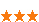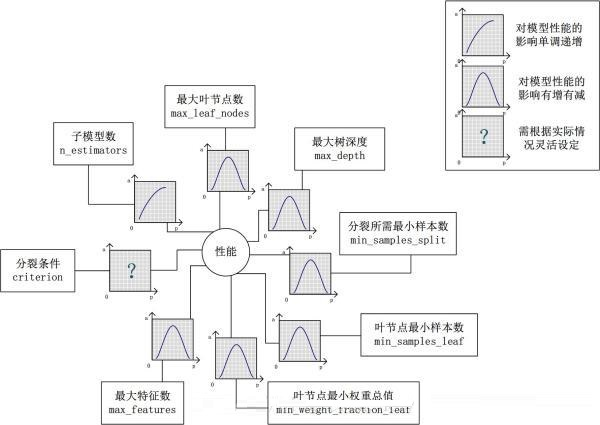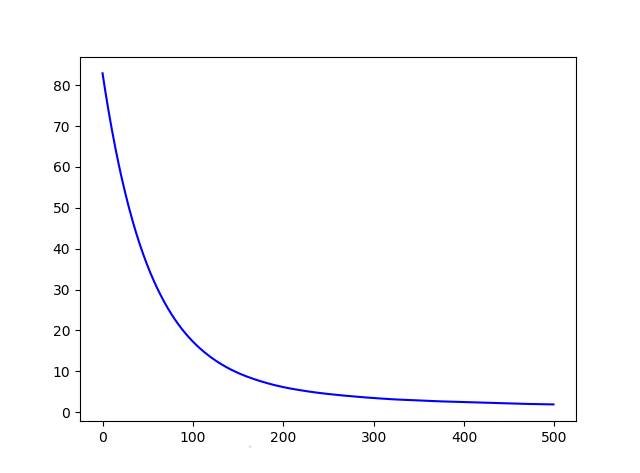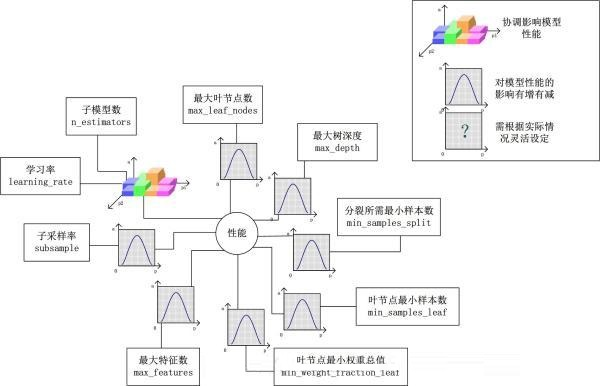您可以捐助，支持我们的公益事业。 1元 10元 50元 认证码：必填求知 文章 文库 Lib 视频 iPerson 课程 认证 咨询 工具 讲座 Modeler Code要资料订阅捐助
python机器学习库sklearn――集成方法2723 次浏览     评价： 好 中 差
2019-7-31

 编辑推荐: 本文来自csdn，本文将介绍sklearn中如何使用集成学习,模块还支持树的并行构建和预测结果的并行计算，希望对您的学习有所帮助。

Bagging 元估计器

 # 产生样本数据集 from sklearn.model_selection import cross_val_score from sklearn import datasets iris = datasets.load_iris() X, y = iris.data[:, 1:3], iris.target# ==================Bagging 元估计器============= from sklearn.ensemble import BaggingClassifier from sklearn.neighbors import KNeighborsClassifier bagging = BaggingClassifier(KNeighborsClassifier(),max_samples=0.5, max_features=0.5) scores = cross_val_score(bagging, X, y) print('Bagging准确率：',scores.mean())

max_samples 和 max_features 控制着子集的大小（对于样例和特征），

bootstrap 和 bootstrap_features 控制着样例和特征的抽取是有放回还是无放回的。

sklearn.ensemble 模块包含两个基于 随机决策树 的平均算法： RandomForest 算法和 Extra-Trees 算法。

 # ==================决策树、随机森林、极限森林对比=============== # 产生样本数据集 from sklearn.model_selection import cross_val_score from sklearn import datasets iris = datasets.load_iris() X, y = iris.data[:, 1:3], iris.target # 决策树 from sklearn.tree import DecisionTreeClassifier clf = DecisionTreeClassifier(max_depth=None, min_samples_split=2,random_state=0) scores = cross_val_score(clf, X, y) print('决策树准确率：',scores.mean()) # 随机森林 from sklearn.ensemble import RandomForestClassifier clf = RandomForestClassifier(n_estimators=10,max_features=2) clf = clf.fit(X, y) scores = cross_val_score(clf, X, y) print('随机森林准确率：',scores.mean()) # 极限随机树 from sklearn.ensemble import ExtraTreesClassifier clf = ExtraTreesClassifier(n_estimators=10, max_depth=None,min_samples_split=2, random_state=0) scores = cross_val_score(clf, X, y) print('极限随机树准确率：',scores.mean()) print('模型中各属性的重要程度：',clf.feature_importances_)

（n_estimators）是森林里树的数量，通常数量越大，效果越好，但是计算时间也会随之增加。 此外要注意，当树的数量超过一个临界值之后，算法的效果并不会很显著地变好。

（max_features）是分割节点时考虑的特征的随机子集的大小。 这个值越低，方差减小得越多，但是偏差的增大也越多。 根据经验，回归问题中使用 max_features = n_features ， 分类问题使用 max_features = sqrt（n_features （其中 n_features 是特征的个数）是比较好的默认值。

max_depth = None 和 min_samples_split = 2 结合通常会有不错的效果（即生成完全的树）。 请记住，这些（默认）值通常不是最佳的，同时还可能消耗大量的内存，最佳参数值应由交叉验证获得。

1、对Random Forest来说，增加“子模型数”（n_estimators）可以明显降低整体模型的方差，且不会对子模型的偏差和方差有任何影响。模型的准确度会随着“子模型数”的增加而提高。由于减少的是整体模型方差公式的第二项，故准确度的提高有一个上限。

2、在不同的场景下，“分裂条件”（criterion）对模型的准确度的影响也不一样，该参数需要在实际运用时灵活调整。

3、调整“最大叶节点数”（max_leaf_nodes）以及“最大树深度”（max_depth）之一，可以粗粒度地调整树的结构：叶节点越多或者树越深，意味着子模型的偏差越低，方差越高；

4、同时，调整“分裂所需最小样本数”（min_samples_split）、“叶节点最小样本数”（min_samples_leaf）及“叶节点最小权重总值”（min_weight_fraction_leaf），可以更细粒度地调整树的结构：分裂所需样本数越少或者叶节点所需样本越少，也意味着子模型越复杂。一般来说，我们总采用bootstrap对样本进行子采样来降低子模型之间的关联度，从而降低整体模型的方差。

5、适当地减少“分裂时考虑的最大特征数”（max_features），给子模型注入了另外的随机性，同样也达到了降低子模型之间关联度的效果。但是一味地降低该参数也是不行的，因为分裂时可选特征变少，模型的偏差会越来越大。在下图中，我们可以看到这些参数对Random Forest整体模型性能的影响：

 # 产生样本数据集 from sklearn.model_selection import cross_val_score from sklearn import datasets iris = datasets.load_iris() X, y = iris.data[:, 1:3], iris.target # ====================AdaBoost========================= from sklearn.ensemble import AdaBoostClassifier clf = AdaBoostClassifier(n_estimators=100) scores = cross_val_score(clf, X, y) print('AdaBoost准确率：',scores.mean())Gradient Tree Boosting 或梯度提升回归树（GBRT）是对于任意的可微损失函数的提升算法的泛化。 GBRT 是一个准确高效的现有程序， 它既能用于分类问题也可以用于回归问题。梯度树提升模型被应用到各种领域，包括网页搜索排名和生态领域。

1) 划分时考虑的最大特征数max_features: 可以使用很多种类型的值，默认是”None”,意味着划分时考虑所有的特征数；如果是”log2”意味着划分时最多考虑logN2log2N个特征；如果是”sqrt”或者”auto”意味着划分时最多考虑N??√N个特征。如果是整数，代表考虑的特征绝对数。如果是浮点数，代表考虑特征百分比，即考虑（百分比x/N）取整后的特征数。其中N为样本总特征数。一般来说，如果样本特征数不多，比如小于50，我们用默认的”None”就可以了，如果特征数非常多，我们可以灵活使用刚才描述的其他取值来控制划分时考虑的最大特征数，以控制决策树的生成时间。

2) 决策树最大深度max_depth: 默认可以不输入，如果不输入的话，决策树在建立子树的时候不会限制子树的深度。一般来说，数据少或者特征少的时候可以不管这个值。如果模型样本量多，特征也多的情况下，推荐限制这个最大深度，具体的取值取决于数据的分布。常用的可以取值10-100之间。

3) 内部节点再划分所需最小样本数min_samples_split: 这个值限制了子树继续划分的条件，如果某节点的样本数少于min_samples_split，则不会继续再尝试选择最优特征来进行划分。 默认是2.如果样本量不大，不需要管这个值。如果样本量数量级非常大，则推荐增大这个值。

4) 叶子节点最少样本数min_samples_leaf: 这个值限制了叶子节点最少的样本数，如果某叶子节点数目小于样本数，则会和兄弟节点一起被剪枝。 默认是1,可以输入最少的样本数的整数，或者最少样本数占样本总数的百分比。如果样本量不大，不需要管这个值。如果样本量数量级非常大，则推荐增大这个值。

5）叶子节点最小的样本权重和min_weight_fraction_leaf：这个值限制了叶子节点所有样本权重和的最小值，如果小于这个值，则会和兄弟节点一起被剪枝。 默认是0，就是不考虑权重问题。一般来说，如果我们有较多样本有缺失值，或者分类树样本的分布类别偏差很大，就会引入样本权重，这时我们就要注意这个值了。

6) 最大叶子节点数max_leaf_nodes: 通过限制最大叶子节点数，可以防止过拟合，默认是”None”，即不限制最大的叶子节点数。如果加了限制，算法会建立在最大叶子节点数内最优的决策树。如果特征不多，可以不考虑这个值，但是如果特征分成多的话，可以加以限制，具体的值可以通过交叉验证得到。

7) 节点划分最小不纯度min_impurity_split: 这个值限制了决策树的增长，如果某节点的不纯度(基于基尼系数，均方差)小于这个阈值，则该节点不再生成子节点。即为叶子节点 。一般不推荐改动默认值1e-7。

 # 产生样本数据集 from sklearn.model_selection import cross_val_score from sklearn import datasets iris = datasets.load_iris() X, y = iris.data[:, 1:3], iris.target # ====================Gradient Tree Boosting （梯度树提升）========================= # 分类 from sklearn.ensemble import GradientBoosting Classifier clf = GradientBoostingClassifier(n_estimators=100, learning_rate=1.0,max_depth=1, random_state=0) scores = cross_val_score(clf, X, y) print('GDBT准确率：',scores.mean()) # 回归 import numpy as np import matplotlib.pyplot as plt from sklearn.metrics import mean_squared_error from sklearn.datasets import load_boston from sklearn.ensemble import GradientBoostingRegressor from sklearn.utils import shuffle from sklearn.model_selection import train_test_split,cross_val_score,cross_validate boston = load_boston() # 加载波士顿房价回归数据集 X1, y1 = shuffle(boston.data, boston.target, random_state=13) # 将数据集随机打乱 X_train, X_test, y_train, y_test = train_test_split (X1, y1, test_size=0.1, random_state=0) # 划分训练集 和测试集.test_size为测试集所占的比例 clf = GradientBoostingRegressor(n_estimators=500, learning_rate=0.01,max_depth=4,min_samples_split=2, loss='ls') clf.fit(X1, y1) print('GDBT回归MSE：',mean_squared_error(y_test, clf.predict(X_test))) # print('每次训练的得分记录：',clf.train_score_) print('各特征的重要程度：',clf.feature_importances_) plt.plot(np.arange(500), clf.train_score_, 'b-') # 绘制随着训练次数增加，训练得分的变化 plt.show()learning_rate 是一个在 (0,1] 之间的超参数，这个参数通过 shrinkage(缩减步长) 来控制过拟合。在训练一定数量的弱分类器时,参数 learning_rate 和参数 n_estimators 之间有很强的制约关系。 较小的 learning_rate 需要大量的弱分类器才能维持训练误差的稳定。经验表明数值较小的 learning_rate 将会得到更好的测试误差。

Least squares ( ‘ls’ ): 由于其优越的计算性能,该损失函数成为回归算法中的自然选择。 初始模型 （校对者注：即损失函数的初始值，下同） 通过目标值的均值给出。

Least absolute deviation ( ‘lad’ ): 回归中具有鲁棒性的损失函数,初始模型通过目 标值的中值给出。

Huber ( ‘huber’ ): 回归中另一个具有鲁棒性的损失函数,它是最小二乘和最小绝对偏差两者的结合. 其利用 alpha 来控制模型对于异常点的敏感度(详细介绍请参考 [F2001]).

Quantile ( ‘quantile’ ): 分位数回归损失函数.用 0 < alpha < 1 来指定分位数这个损 失函数可以用来产生预测间隔。（详见 Prediction Intervals for Gradient Boosting Regression ）。

Binomial deviance (‘deviance’): 对于二分类问题(提供概率估计)即负的二项 log 似然损失函数。模型以 log 的比值比来初始化。

Multinomial deviance (‘deviance’): 对于多分类问题的负的多项log似然损失函数具有 n_classes 个互斥的类。提供概率估计。 初始模型由每个类的先验概率给出.在每一次迭代中 n_classes 回归树被构建,这使得 GBRT 在处理多类别数据集时相当低效。

Exponential loss (‘exponential’): 与 AdaBoostClassifier 具有相同的损失函数。与 ‘deviance’ 相比，对被错误标记的样本的鲁棒性较差，仅用于在二分类问题。

GDBT调参策略：Voting Classifier（投票分类器）

VotingClassifier （投票分类器）的原理是结合了多个不同的机器学习分类器,并且采用多数表决（majority vote）（硬投票） 或者平均预测概率（软投票）的方式来预测分类标签。 这样的分类器可以用于一组同样表现良好的模型,以便平衡它们各自的弱点。

 # 产生样本数据集 from sklearn.model_selection import cross_val_score from sklearn import datasets iris = datasets.load_iris() X, y = iris.data[:, 1:3], iris.target # ====================Voting Classifier（投票分类器）========================= from sklearn.linear_model import LogisticRegression from sklearn.naive_bayes import GaussianNB from sklearn.ensemble import RandomForestClassifier from sklearn.ensemble import VotingClassifier clf1 = LogisticRegression(random_state=1) clf2 = RandomForestClassifier(random_state=1) clf3 = GaussianNB() eclf = VotingClassifier(estimators=[('lr', clf1), ('rf', clf2), ('gnb', clf3)], voting='hard') # 无权重投票 eclf = VotingClassifier(estimators=[('lr', clf1), ('rf', clf2), ('gnb', clf3)],voting='soft', weights=[2,1,2]) # 权重投票 for clf, label in zip([clf1, clf2, clf3, eclf], ['Logistic Regression', 'Random Forest', 'naive Bayes', 'Ensemble']): scores = cross_val_score(clf,X,y,cv=5, scoring='accuracy') print("准确率: %0.2f (+/- %0.2f) [%s]" % (scores.mean(), scores.std(), label)) # 配合网格搜索 from sklearn.model_selection import GridSearchCV params = {'lr__C': [1.0, 100.0], 'rf__n_estimators': [20, 200],} # 搜索寻找最优的lr模型中的C参数和rf模型中的n_estimators grid = GridSearchCV(estimator=eclf, param_grid=params, cv=5) grid = grid.fit(iris.data, iris.target) print('最优参数：',grid.best_params_)2723 次浏览     评价： 好 中 差订阅捐助
 相关文章 我们该如何设计数据库 数据库设计经验谈 数据库设计过程 数据库编程总结
 相关文档 数据库性能调优技巧 数据库性能调整 数据库性能优化讲座 数据库系统性能调优系列